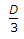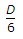# Civil Engineering - Estimating and Costing - Discussion

### Discussion :: Estimating and Costing - Section 1 (Q.No.4)

4.

The correct prismoidal formula for volume is

 [A]. D [first area + last area + ∑ Even area + 2 ∑ odd areas] [B].[first area + last area + 4 ∑ Even area + 2 ∑ odd areas] [C].[first area + last area + 2 ∑ Even area + 4 ∑ odd areas] [D].[first area + last area + 2 ∑ Even area + 4 ∑ odd areas].

Explanation:

No answer description available for this question.

 A Arul said: (Jun 27, 2015) Kindly give answers with full explanation its useful to all.

 Mudasir said: (Sep 9, 2015) Give me answer.

 Sukhamoy said: (Oct 2, 2015) (L/3) should be multiplied with option B, where L is the total length of strip.

 Rukshana said: (Jun 24, 2016) Please explain the full answer with correct explanation.

 Raja said: (Aug 9, 2016) Please give me a perfect explanation.

 Md Saif said: (Nov 18, 2016) Please give me a proper explanation.

 Yuvaraj Kirupakaran said: (Jan 4, 2017) Please give me a full explanation.

 Kamruz said: (Jan 5, 2017) I think C is correct.

 Ashok said: (Jan 26, 2017) Please give the explanation of the answer.

 Deepak said: (Feb 24, 2017) Option C is the Right Answer.

 S.Shahin said: (Apr 9, 2017) B is correct. Because here even means v=L/3{ A.+An+4(A1+A3+.....+An-1)+2(A2+A4+......+An-2)} Here, A = Coross sectional Area.

 Somya said: (Jun 19, 2017) B is correct according to Simpson's 1/3rd formula.

 Ps111 said: (Dec 14, 2017) C is correct. D/3 *[(1ST AREA+LAST AREA) + 4*(ODD)+ 2*(EVEN)].

 Suryashekhar Vicky said: (Jan 5, 2018) option C is right. Ref: B.N Dutt book.

 Pratik Soni said: (Jan 14, 2018) According to B. N. Dutta "C" is correct Answer.

 Rangareddy said: (Jan 31, 2018) As per my knowledge, option 3 is correct.

 Amz said: (Feb 28, 2018) None of the above. The question lacks explanation of D, presumably equal spacing of areas. Prismoidal formula treats areas as vector ordinates and averages the areas. In the same way the area of a prism=median area (Top+Bottom)/2*height. V = D/2*[V1 + Vn + 2* (V2+V3+..+Vn-2)].

 Rishabh Machiwal said: (Mar 8, 2018) Both options B and C are right. If we take A1, A2, A3.......,An-1, An then option B is right. If we take A0, A1, A2, A3.....An-1, An then option C is right.

 Anju said: (Apr 4, 2018) B is the right option. I agree.

 Lalan said: (May 18, 2018) Yes, @Rishabh. In both case C is correct.

 Ashish said: (Jun 6, 2018) The answer is C.

 Ruata said: (Oct 2, 2018) Option C is the correct answer.

 Ashtaveer said: (Oct 20, 2018) Prismodal formula = h/6( A1+A2 +4Am) where Am=(A1+A2)/2.

 Aman said: (Nov 21, 2018) L/3 (1st area + last area + 4 (sum of even area) + 2 (sum of odd area). It's Simson rule.

 Indhuca said: (Jun 21, 2019) => [first area + last area + 4 Σ Even area + 2 Σ odd areas is the Right answer and it is Also Known as Simpson Rule.

 Dayananda Naik T L said: (Aug 1, 2020) I think C is the correct answer.

 Rekha said: (Dec 5, 2020) @Indhuca. Computation of area - Simpson's rule. Computation of volume- Prismoidal formula. Both equations are same.

 Alfaizal said: (Jan 4, 2021) B is the right answer I agree.